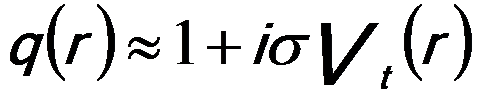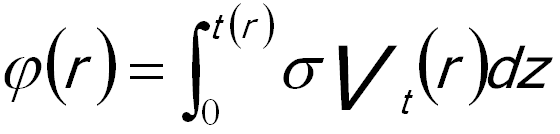Weak-Phase-Objects (WPO) in TEM Observations
- Practical Electron Microscopy and Database -
- An Online Book -

https://www.globalsino.com/EM/

 ================================================================================= The TEM sample is usually described by a projected potential model where the atomic potentials will modify the phase (and only the phase) of the wave of the incident electrons as the electrons passes through the material, which is represented by the phase object approximation (POA), given by,------------------- [4173a] where,          σ -- An interaction constant,          Vt -- The projected potential of the specimen. When the specimen is thin, σVt is much less than unity and thus the phase change is small. By using the first-degree Taylor polynomial of q(r), the POA model can be further simplified by the weak phase object approximation (WPOA), which is a linear approximation, given by,------------------- [4173b] The assumption that σVt is small holds for many thin TEM specimens. However, it fails for electrons even passing through a single uranium atom. The information about the specimen potential is contained in the phase shift function through the relation,------------------- [4173c] Here, t(r) is the local thickness of the TEM specimen, and the electron beam is traveling in the +z direction. Weak-phase-objects (WPO) scatter electrons weakly. These objects can be, for instance, thin amorphous specimens and very thin crystalline specimens (especially if tilted slightly from the zone-axis condition ). In the weak phase case, the electron beam passing thorough the object (thin specimen) only suffers a modest phase shift, but its amplitude is effectively unchanged. The weak phase object approximation assumes that the phase change introduced by the crystal potential is so small that a first order expansion may be applied to simply the form of the exit wave (see Equations 4217). Note that for the weak phase object approximation the diffractogram obtained using EFG-TEMs is the same as that using a CTEM based on W (tungsten) or LaB6 electron guns. Information limit for HRTEMs is the inverse of the maximum spatial object frequency. The information limit is often obtained from measurements of diffractogram or from Young’s fringes provided a weak phase object scattering beyond the inverse of the information limit. The structure factor F(u) is proportional to the Fourier components Iim(u) of the HREM image. For a weak-phase-object, the Fourier transform of the HREM image Iim(u) can be given by ,------------------- [4173d] where,          k’ -- A constant          T(u) -- The contrast transfer function (CTF) Gibson  proposed that in the weak-phase object approximation, the TEM image intensity at ~1 nm resolution is given by,------------------- [4173e] where,          I0 -- The main beam intensity,          φ(r) -- The phase shift caused by the TEM specimen,-- Represents a convolution integral,          FT -- Represents a Fourier transform,          K -- The reciprocal space coordinate,          χ -- The phase shift function,          P(K) -- The damping envelope caused by microscope instabilities and limited coherence. Assuming the electron beam is a Gaussian-shaped source, a high-frequency damping function on TEM images can be approximately given by,-------------------- [4173f] where,          Δ -- The defocus spread due to a nonmonochromatic beam and electronic instabilities,          λ -- The wavelength of the electron beam,          k -- Spatial frequency,          θc -- The beam convergence angle,          Δf -- The defocus,                    Cs -- Spherical aberration of the objective lens. The significant dependence on wavevector leads to a serious damping envelope, and slight changes in operating conditions can induce extreme changes in the contrast transfer. All the purpose of the methods of the electron image series reconstructions with TEM is to find an object wave function, ψo,s. The reconstruction methods can be basically categorized by two groups: i) weak phase object approximation (WPOA) and more generally ii) phase object approximation (POA). In principle, the methods based on the POA give a better estimation of the object wave function, but they don’t have closed form solutions and have to be computed iteratively and thus is very time consuming.  On the other hand, the reconstruction methods based on WPOA are fast but the TEM imaging requires much thinner samples.          M.A. OKeefe, V. Radmilovic, Proceedings of the XIIIth International Congress for Electron Microscopy, Vol. 1, 1994, pp. 361–362.  X. D. Zou. Crystal Structure determination by crystallographic image processing. in “Electron Crystallography”, eds. D. L. Dorset, S. Hovmöller, X. D. Zou, Nato ASI Series C, Kluwer Academic Publishers, Dordrecht, 1997, pp163-181.  A. I. Kirkland and R. R. Meyer, Indirect high resolution transmission electron microscopy: Aberration measurement and wavefunction reconstruction, Microscopy and Microanalysis, vol. 10, pp. 401–413, 2004.  J.M. Gibson, Ultramicroscopy 56 (1994) 26.
=================================================================================